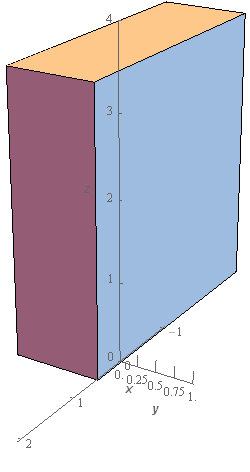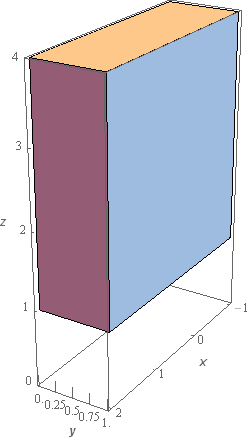Paul's Online Notes
Home / Calculus III / Surface Integrals / Divergence Theorem
Show Mobile Notice Show All Notes Hide All Notes
Mobile Notice
You appear to be on a device with a "narrow" screen width (i.e. you are probably on a mobile phone). Due to the nature of the mathematics on this site it is best views in landscape mode. If your device is not in landscape mode many of the equations will run off the side of your device (should be able to scroll to see them) and some of the menu items will be cut off due to the narrow screen width.

### Section 17.6 : Divergence Theorem

2. Use the Divergence Theorem to evaluate $$\displaystyle \iint\limits_{S}{{\vec F\centerdot d\vec S}}$$ where $$\vec F = \sin \left( {\pi x} \right)\,\vec i + z{y^3}\,\vec j + \left( {{z^2} + 4x} \right)\,\vec k$$ and $$S$$ is the surface of the box with $$- 1 \le x \le 2$$, $$0 \le y \le 1$$ and $$1 \le z \le 4$$. Note that all six sides of the box are included in $$S$$.

Show All Steps Hide All Steps

Start Solution

Let’s start off with a quick sketch of the surface we are working with in this problem.We included a sketch with traditional axes and a sketch with a set of “box” axes to help visualize the surface.

Note as well here that because we are including all six sides of the box shown above that the surface does enclose (or is the boundary curve if you want to use that terminology) for the box.

Show Step 2

We are going to use the Divergence Theorem in the following direction.

$\iint\limits_{S}{{\vec F\centerdot d\vec S}} = \iiint\limits_{E}{{{\mathop{\rm div}\nolimits} \vec F\,dV}}$

where $$E$$ is just the solid shown in the sketches from Step 1.

$$E$$ is just a box and the limits defining it where given in the problem statement. The limits for our integral will then be,

$\begin{array}{c} - 1 \le x \le 2\\ 0 \le y \le 1\\1 \le z \le 4\end{array}$

We’ll also need the divergence of the vector field so here is that.

${\mathop{\rm div}\nolimits} \vec F = \frac{\partial }{{\partial x}}\left( {\sin \left( {\pi x} \right)} \right) + \frac{\partial }{{\partial y}}\left( {z{y^3}\,} \right) + \frac{\partial }{{\partial z}}\left( {{z^2} + 4x} \right) = \pi \cos \left( {\pi x} \right) + 3z{y^2} + 2z$ Show Step 3

Now let’s apply the Divergence Theorem to the integral and get it converted to a triple integral.

\begin{align*}\iint\limits_{S}{{\vec F\centerdot d\vec S}} & = \iiint\limits_{E}{{{\mathop{\rm div}\nolimits} \vec F\,dV}}\\ & = \iiint\limits_{E}{{\pi \cos \left( {\pi x} \right) + 3z{y^2} + 2z\,dV}}\\ & = \int_{{ - 1}}^{2}{{\int_{0}^{1}{{\int_{1}^{4}{{\pi \cos \left( {\pi x} \right) + 3z{y^2} + 2z\,dz}}\,dy}}\,dx}}\end{align*} Show Step 4

All we need to do then in evaluate the integral.

\begin{align*}\iint\limits_{S}{{\vec F\centerdot d\vec S}} & = \int_{{ - 1}}^{2}{{\int_{0}^{1}{{\int_{1}^{4}{{\pi \cos \left( {\pi x} \right) + 3z{y^2} + 2z\,dz}}\,dy}}\,dx}}\\ & = \int_{{ - 1}}^{2}{{\int_{0}^{1}{{\left. {\left( {\pi z\cos \left( {\pi x} \right) + \frac{3}{2}{z^2}{y^2} + {z^2}} \right)} \right|_1^4\,dy}}\,dx}}\\ & = \int_{{ - 1}}^{2}{{\int_{0}^{1}{{3\pi \cos \left( {\pi x} \right) + \frac{{45}}{2}{y^2} + 15\,dy}}\,dx}}\\ & = \int_{{ - 1}}^{2}{{\left. {\left( {3y\pi \cos \left( {\pi x} \right) + \frac{{15}}{2}{y^3} + 15y} \right)} \right|_0^1\,dx}}\\ & = \int_{{ - 1}}^{2}{{3\pi \cos \left( {\pi x} \right) + \frac{{45}}{2}\,dx}}\\ & = \left. {\left( {3\sin \left( {\pi x} \right) + \frac{{45}}{2}x} \right)} \right|_{ - 1\pi }^2 = \require{bbox} \bbox[2pt,border:1px solid black]{{\frac{{135}}{2}}}\end{align*}